# AP Calculus AB : Antiderivatives following directly from derivatives of basic functions

## Example Questions

### Example Question #11 : Techniques Of Antidifferentiation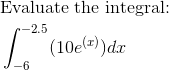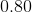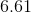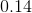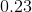Explanation: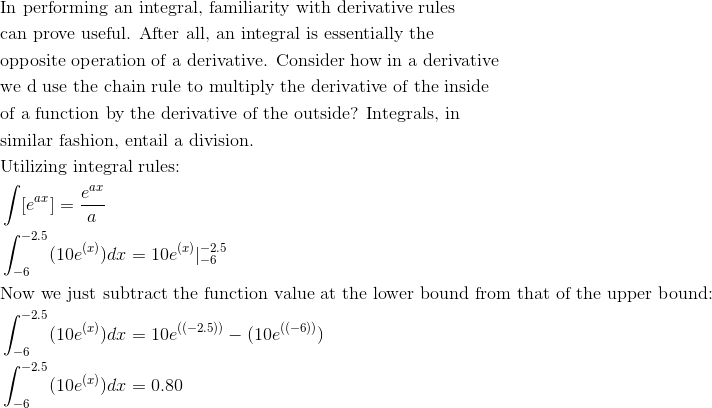### Example Question #12 : Techniques Of Antidifferentiation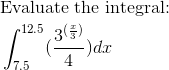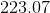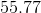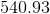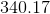Explanation: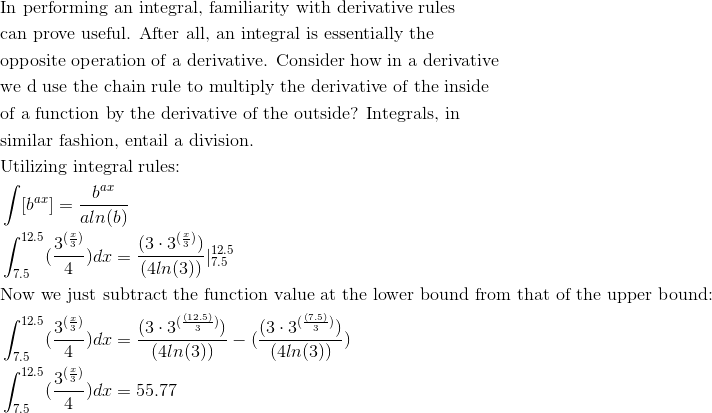### Example Question #13 : Techniques Of Antidifferentiation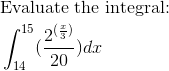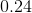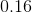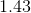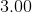Explanation: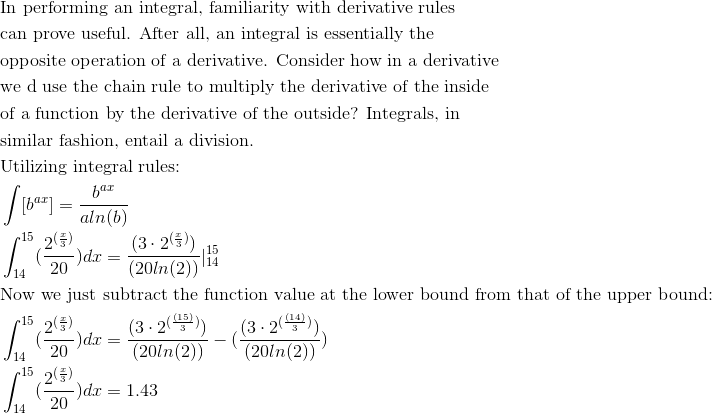### Example Question #14 : Techniques Of Antidifferentiation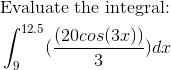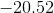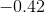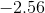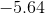Explanation: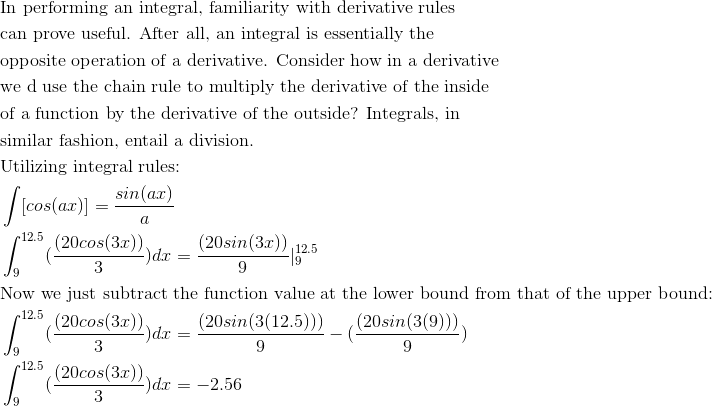### Example Question #81 : Integrals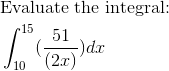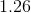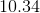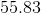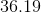Explanation: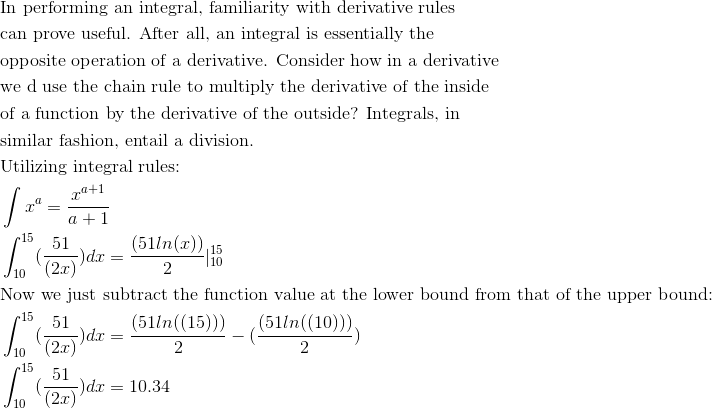### Example Question #21 : Techniques Of Antidifferentiation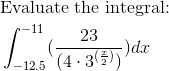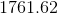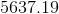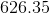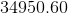Explanation: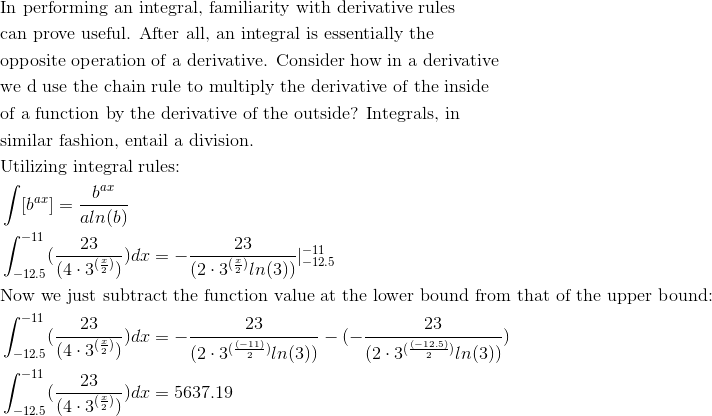### Example Question #22 : Techniques Of Antidifferentiation

Given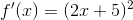, find the general form for the antiderivative.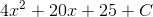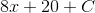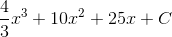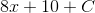Explanation:

To answer this, we will need to FOIL our function first.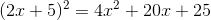Now can find the antiderivatives of each of these three summands using the power rule.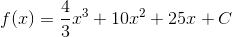(Don't forget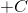)!

### Example Question #23 : Techniques Of Antidifferentiation

Compute the following integral: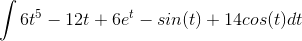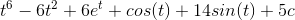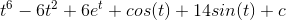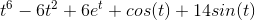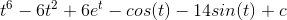Explanation:

Compute the following integral:Now, we need to recall a few rules.

1)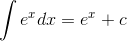2)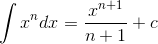3)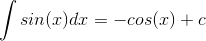4)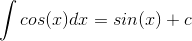We can use all these rules to change our original function into its anti-derivative.We can break this up into separate integrals for each term, and apply our rules individually.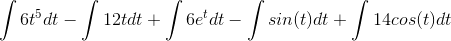The first two integrals can be found using rule 2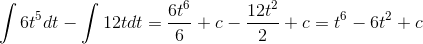Next, let's tackle the middle integral: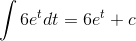Then the "sine" integral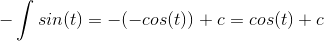And finally, the cosine integral.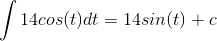Now, we can put all of this together to get:Note that we only have 1 c, because the c is just a constant.

### Example Question #24 : Techniques Of Antidifferentiation

Solve: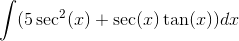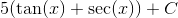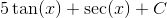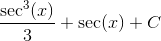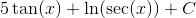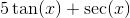Explanation:

The integral can be solved knowing the derivatives of the following functions: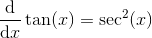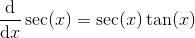Given that the integrand is simply the sum of these two derivatives, we find that our integral is equal to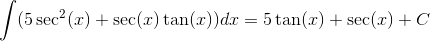### Example Question #25 : Techniques Of Antidifferentiation

Solve: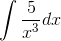None of the other answers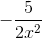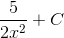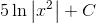None of the other answers

Explanation:

The integral is equal to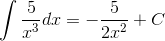and was given by the following rule: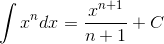Using this rule becomes more clear when we rewrite the integral as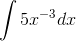Note that because none of the answer choices had the integration constant C along with the proper integral result, the correct choice was "None of the other answers." Always check after solving an indefinite integral for C!